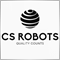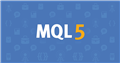# Add trailing stop in EA46

Hi everyone,

I have already been helped by members of mql5, so I try again with an other problem.

I want to add Trailing stop in my EA but I don't know where I can put the modification without break my code.

For exemple, this is my part of 'Order Close - Buy' :

```
double SL_Buy = NormalizeDouble(Bid- StopLoss * Point, Digits);
double TP_Buy = NormalizeDouble(Bid+ TakeProfit * Point, Digits);

------------------------
for (i = OrdersTotal(); i >= 0; i--) {

// if the candle 0 (Close ) is lower than the candle 2 (Close)
// if the RSI  is lower than 48

if (OrderSelect(OrderTicket(), SELECT_BY_TICKET, MODE_TRADES) == true)
{
if (OrdersTotal() >= 1 && OrderType() == OP_BUY && RSI1 <= 45)
{
}
} ```

And I want to add this type of trailing stop (activate for breakeven) :

```int trailing_stop = 20;
double ts;

for (int i = OrdersTotal()-1; i >= 0; i --)
{
if (OrderSymbol() == Symbol())
{
{
ts = Bid-(Point*trailing_stop);
if (OrderStopLoss()=(Point*trailing_stop))
OrderModify(OrderTicket(),OrderOpenPrice(),ts,OrderTakeProfit(),0,White);```

If someone can help me :)714819

jonathan75:

Hi everyone,

I have already been helped by members of mql5, so I try again with an other problem.

I want to add Trailing stop in my EA but I don't know where I can put the modification without break my code.

For exemple, this is my part of 'Order Close - Buy' :

And I want to add this type of trailing stop (activate for breakeven) :

If someone can help me :)

```int trailing_stop = 20;
double ts;

for (int i = OrdersTotal()-1; i >= 0; i --)
{
if (OrderSymbol() == Symbol())
{
{
ts = Bid-(Point*trailing_stop);
if (OrderStopLoss()=(Point*trailing_stop))
OrderModify(OrderTicket(),OrderOpenPrice(),ts,OrderTakeProfit(),0,White);

//-------------->try this<-------------------------------------------------
int trailing_stop = 20;
double ts;
void OnTick()
{
if(trailing_stop >0)
Trail(trailing_stop);
return;
}

void Trail(int _stop)//Put this function at the end of your code
{
for (int i = OrdersTotal()-1; i >= 0; i --)
{
if (OrderSymbol() == Symbol())
{
{
ts = Bid-(Point*_stop);
if (OrderStopLoss()=(Point*_stop))
OrderModify(OrderTicket(),OrderOpenPrice(),ts,OrderTakeProfit(),0,White);
}```

Try my example

The void Trail function you can put at the end of your code and then call it on tickDocumentation on MQL5: Constants, Enumerations and Structures / Named Constants / Predefined Macro Substitutions
• www.mql5.com
//| Expert initialization function                                   | //| Expert deinitialization function                                 | //| Expert tick function                                             | //| test1                                                            |...